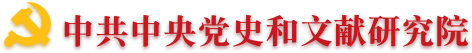•• 理論中國網   |
•"黨的文獻"公眾號"黃城根下"公眾號"黨的歷史"公眾號
•（微博）黨史網1993年被評為編審，並獲國務院政府特殊津貼。曾被聘為中共中央文獻研究會名譽理事、毛澤東思想生平研究分會名譽常務理事。在文獻研究室工作期間，先后參加編輯《毛澤東農村調查文集》、《毛澤東書信選集》、《毛澤東早期文稿》，擔任《建國以來毛澤東文稿》、《毛澤東選集》第二版、《毛澤東文集》、《毛澤東著作專題摘編》等多部著作的主編之一,並擔任大型畫冊《毛澤東》的副主編，大型電視文獻紀錄片《毛澤東》的總監制之一。曾參與《毛澤東軍事文集》、《緬懷毛澤東》、《毛澤東讀書筆記》等著作，以及有關毛澤東的大量文稿的審稿工作。其間曾撰寫多篇論文，參加中央宣傳部文獻研究室等單位舉辦的學術研討會。在紀念毛澤東誕生120周之際，曾以《毛澤東思想當代價值論集》為名結集出版。

• 翻譯
• C
• D
• F
• G
• H
• J
• L
• M
• O
• P
• Q
• S
• T
• W
• X
• Y
• Z
• C
• F
• G
• H
• J
• L
• P
• Q
• S
• T
• W
• X
• Y
• Z
• C
• D
• G
• H
• J
• L
• Q
• S
• W
• X
• Y
• Z